University of Minnesota http://www.umn.edu/ 612-625-5000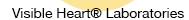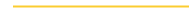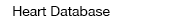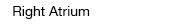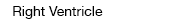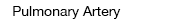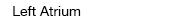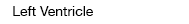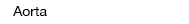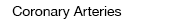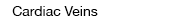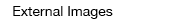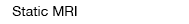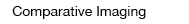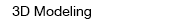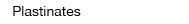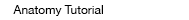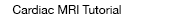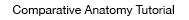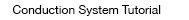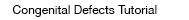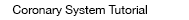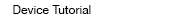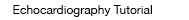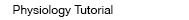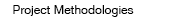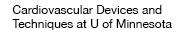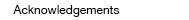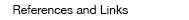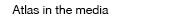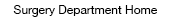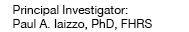The task of maintaining an adequate interstitial homeostasis (the proper nutritional environment surrounding all cells in your body) requires that blood flows almost continuously through each of the millions of capillaries in the body. The following is a brief description of the parameters that govern flow through a given vessel. All bloods vessels have certain lengths (L) and internal radii (r) through which blood flows when the pressure in the inlet and outlet are unequal (Pi and Po respectively); in other words there is a pressure difference (ΔP) between the vessel ends, which supplies the driving force for flow. Because friction develops between moving blood and the stationary vessels walls, this fluid movement has a given resistance (vascular), which is the measure of how difficult it is to move blood through a vessel. One can then describe a relative relationship between vascular flow, the pressure difference, and resistance (i.e., the basic flow equation):

 Flow = pressure differenceresistances or Q = ΔPR
where Q = flow rate (volume/time); ΔP = pressure difference (mm Hg); and R = resistance to flow (mm Hg x time/volume).

This equation may be applied not only to a single vessel, but can also be used to describe flow through a network of vessels (i.e., the vascular bed of an organ or the even your entire systemic circulatory system). It is known that the resistance to flow through a cylindrical tube or vessel depends on several factors (described by Poiseuille) including: 1) radius, 2) length, 3) viscosity of the fluid (blood), and 4) inherent resistance to flow, as follows:

 R = 8 L ηπ r4
where r = inside radius of the vessel, L = vessel length, and η = blood viscosity.

It is important to note that a small change in vessel radius will have a very large influence (4th power) on its resistance to flow; e.g., decreasing vessel diameter by 50% will increase its resistance to flow by approximately 16 fold.

If one combines the preceding two equations into one expression, which is commonly known as the Poiseuille equation, it can be used to better approximate the factors that influence flow though a cylindrical vessel:

 Q = ΔP π r48 L η

Importantly, flow will only occur when a pressure difference exists. Hence, it is not surprising that arterial blood pressure is perhaps the most regulated cardiovascular variable in the human body, and this is principally accomplished by regulating the radii of vessels (e.g., arterioles and metarterioles) within a given tissue or organ system. Whereas vessel length and blood viscosity are factors that influence vascular resistance, they are not considered variables that can be easily regulated for the purpose of the moment-to-moment control of blood flow. Regardless, the primary function of the heart is to keep pressure within arteries higher than those in veins, hence to create a pressure gradient to induce flow. Normally, the average pressure in systemic arteries is approximately 100 mm Hg, and which decreases to near 0 mm Hg in the great caval veins (superior and inferior vena cavae).

The volume of blood that flows through any tissue in a given period of time (normally expressed as mL/min) is called the local blood flow. The velocity (speed) of blood flow (expressed as cm/sec) can generally be considered to be inversely related to the vascular cross-sectional area, such that velocity is slowest where the total cross-sectional area is largest.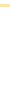© 2021 Regents of the University of Minnesota. All rights reserved. The University of Minnesota is an equal opportunity educator and employer. Privacy Statement